# Average rotation

of the argument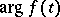of a complex-valued, uniformly almost-periodic functionA phenomenon consisting of the existence (given certain conditions, see below) of the limit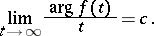The limit itself is also called the average rotation (mean motion). If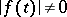for all, then the selection of a continuous branch ofis implied. For analytic almost-periodic functions, the concept of average rotation can be retained even whencontains zeros. Namely, the concepts of "right" and "left" arguments are introduced, whose difference jumps byat a zero of multiplicityof, and one therefore also speaks of right and left average rotation, unless they coincide, in which case one simply speaks of average rotation.

The question of the average rotation arose in connection with the fact that in celestial mechanics the longitude of the perihelion of a planet is expressed approximately as the argument of a certain trigonometric polynomial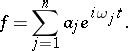J.L. Lagrange studied two simple cases, namely, where one of the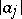is greater than the sum of the remaining coefficients, and for; he noted that in other cases the question is complicated. The study of this question has been taken up only in the 20th century (for its history, see ). The final result that a trigonometric polynomial always has an average rotation was stated in 1938 by B. Jessen (for its proof, see ). (From the point of view of the theory of dynamical systems, it is a matter of averaging a certain function on a torus along the trajectories of the flow defined by shifts by elements of a one-parameter subgroup. However, this function has singularities that obstruct the automatic use of the corresponding general theorem.) Even earlier than this, H. Bohr proved the existence of an average rotation for any uniformly almost-periodic function for which(see ). In this case, the differenceis a uniformly almost-periodic function and is bounded. The average rotation of analytic almost-periodic functions in the general case has also been studied (see , ). In this case, the average rotation does not always exist, but if it does, then the differenceis not necessarily bounded. Nonetheless, it can still possess certain generalized properties of almost-periodicity; this is true, in particular, for trigonometric polynomials . Except in the analytic case, only isolated results concerning the average rotation of a functionfor which,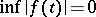, exist (see , ).